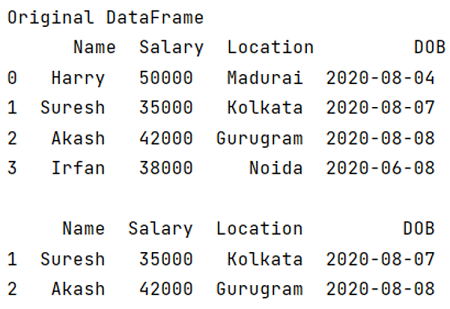# How to select DataFrame rows between two dates?

Learn how to select DataFrame rows between two dates? By Pranit Sharma Last updated : September 20, 2023

Pandas is a special tool which allows us to perform complex manipulations of data effectively and efficiently. Pandas is a vast library of thousands of amazing methods including various data structures, numerical data and datetime.

The datetime is a library in python which is a collection of date and time. Inside Datetime, we can access date and time in any format, but usually date is present in the format of "yy-mm-dd".

Here,

• yy means year
• mm means month
• dd means day

## Problem statement

Given a Pandas DataFrame, we have to select DataFrame rows between two dates.

## Selecting DataFrame rows between two dates

For this purpose, we will just simply compare the dates and filter our rows using pandas.DataFrame.loc Property. It is used to access a group of rows and columns by label(s) or a boolean array.

Note

To work with pandas, we need to import pandas package first, below is the syntax:

```import pandas as pd
```

Let us understand with the help of an example.

## Python program to select DataFrame rows between two dates

```# Importing pandas package
import pandas as pd

# Creating a dictionary
data = {
'Name': ['Harry', 'Suresh','Akash', 'Irfan'],
'Salary': [50000, 35000, 42000, 38000],
'DOB': ['2020-08-04', '2020-08-07', '2020-08-08', '2020-06-08']
}

# Creating DataFrame
df = pd.DataFrame(data)

# Display original DataFrame
print("Original DataFrame\n",df,"\n")

# Filtering rows based on comparison of dates,
# for this purpose we need two random dates
date1 = '2020-08-05'
date2 = '2020-08-08'

result = (df['DOB'] > date1) & (df['DOB'] <= date2)

# Selecting rows from DataFrame
df = df.loc[result]

# Display Result
print(df)
```

### Output

The output of the above program is: